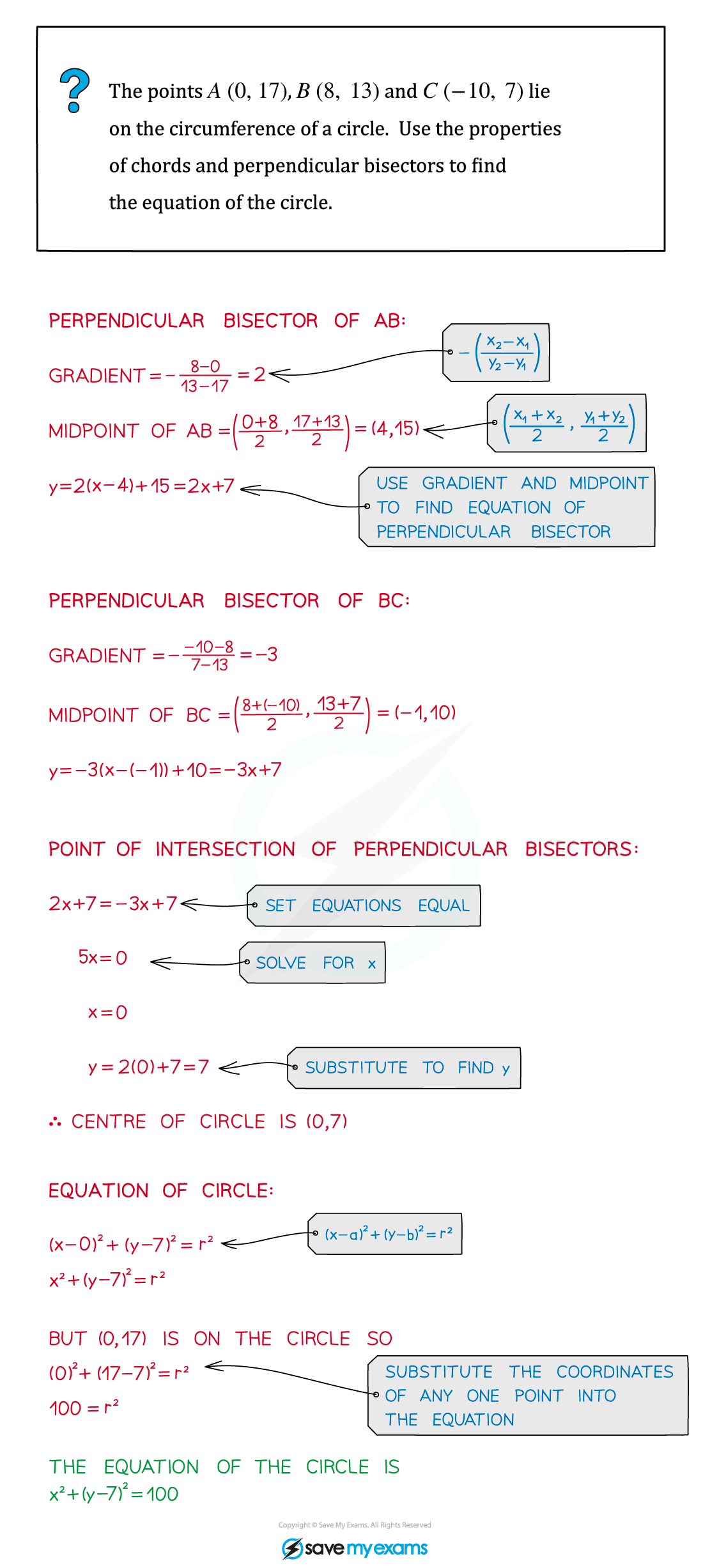# AQA A Level Maths: Pure复习笔记3.2.3 Bisection of Chords

### Bisection of Chords

#### How can I find the equation of a perpendicular bisector?

• The perpendicular bisector of a line segment:
• is perpendicular to the line segment
• goes through the midpoint of the line segment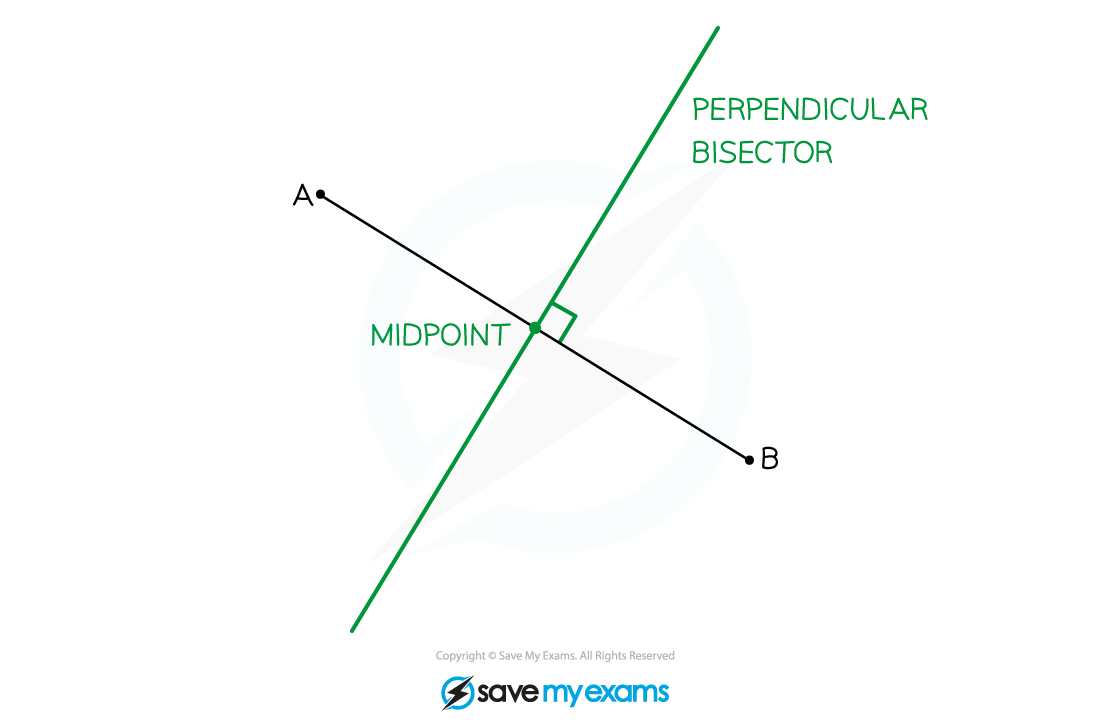•  The midpoint and gradient of the line segment between points (x1, y1) and (x2, y2) are given by the formulae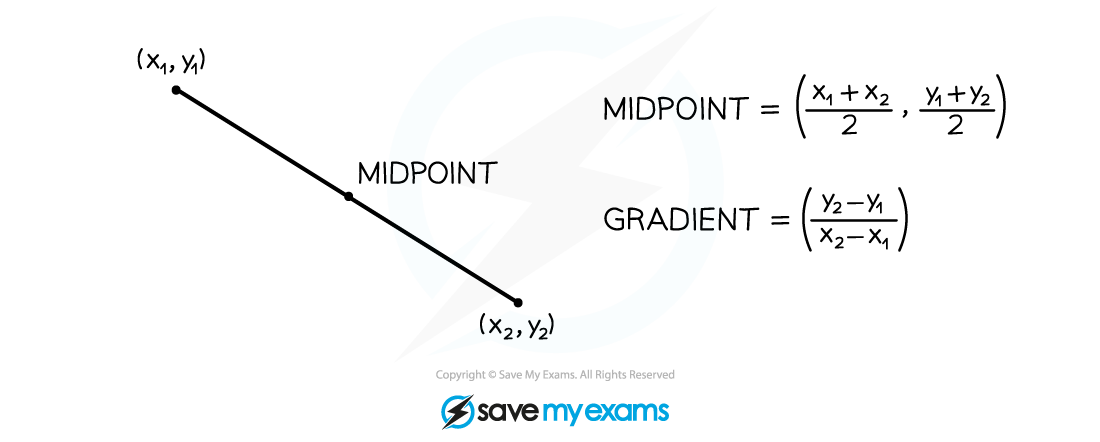•  The gradient of the perpendicular bisector is therefore• The equation of the perpendicular bisector is the equation of the line with that gradient through the line segment's midpoint (see Equation of a Straight Line)

#### How can I use perpendicular bisectors to find the equation of a circle?

• A chord of a circle is a straight line segment between any two points on the circle• The perpendicular bisector of a chord always goes through the centre of the circle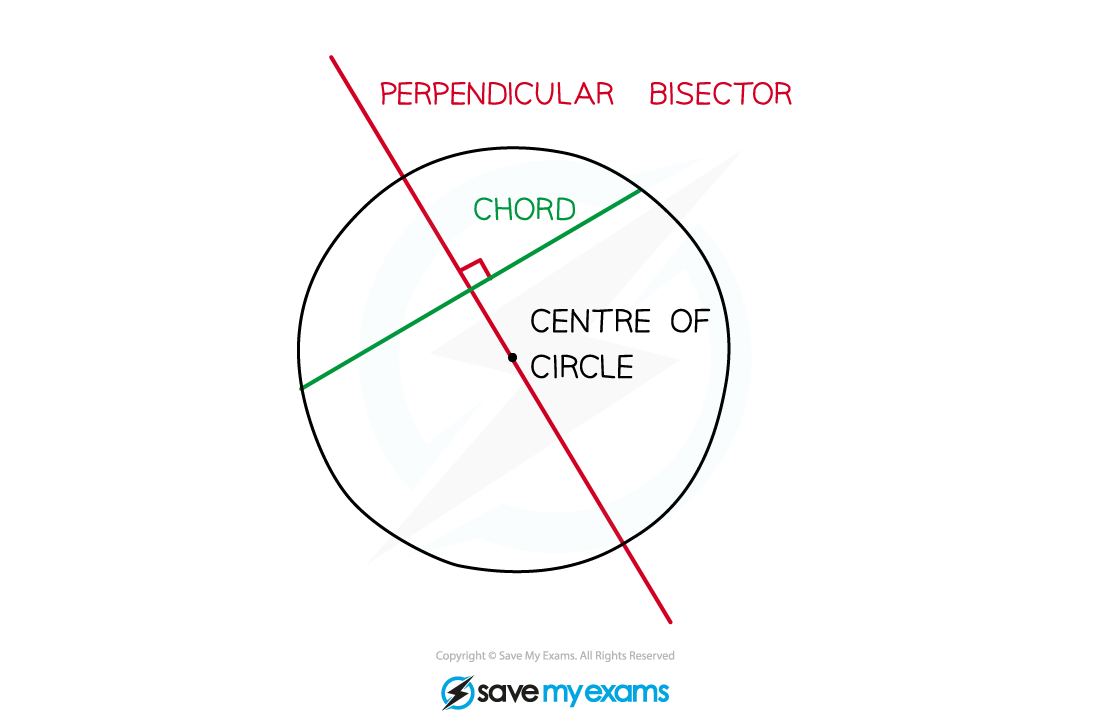• If you know three points on a circle, draw two chords between them – the perpendicular bisectors of the chords will meet at the centre of the circle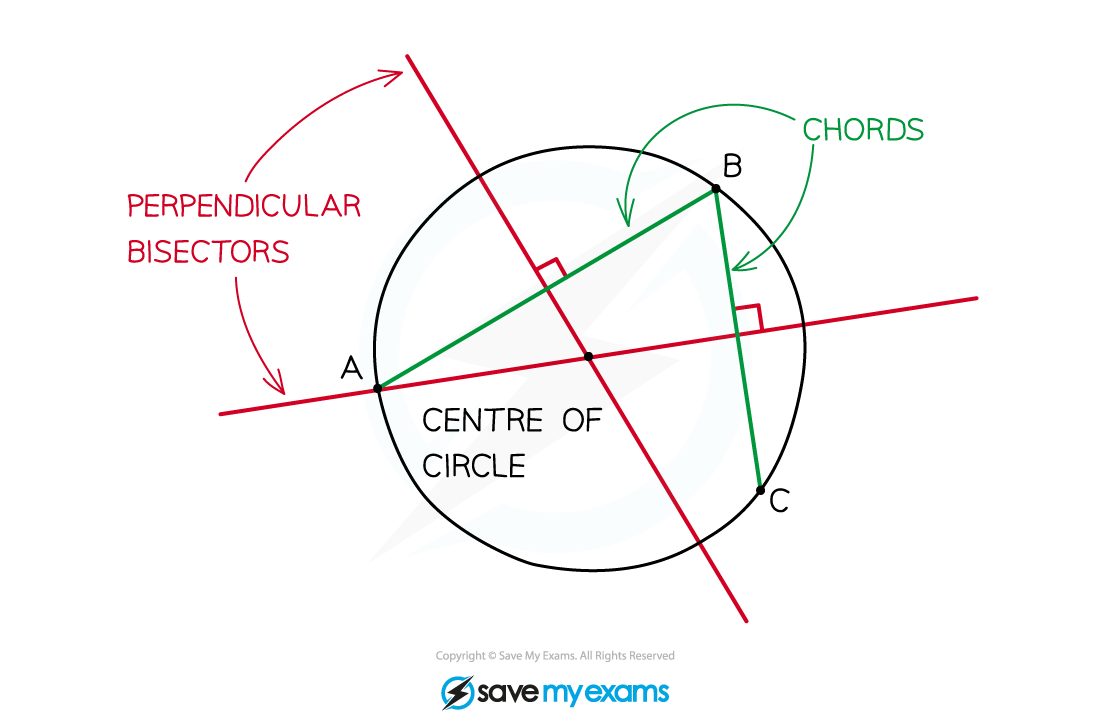• Now that you know the centre of the circle and a point on the circle you can write the equation of the circle

#### Exam Tip

• To find the point of intersection of two straight lines, set the equations of the lines equal to each other and solve.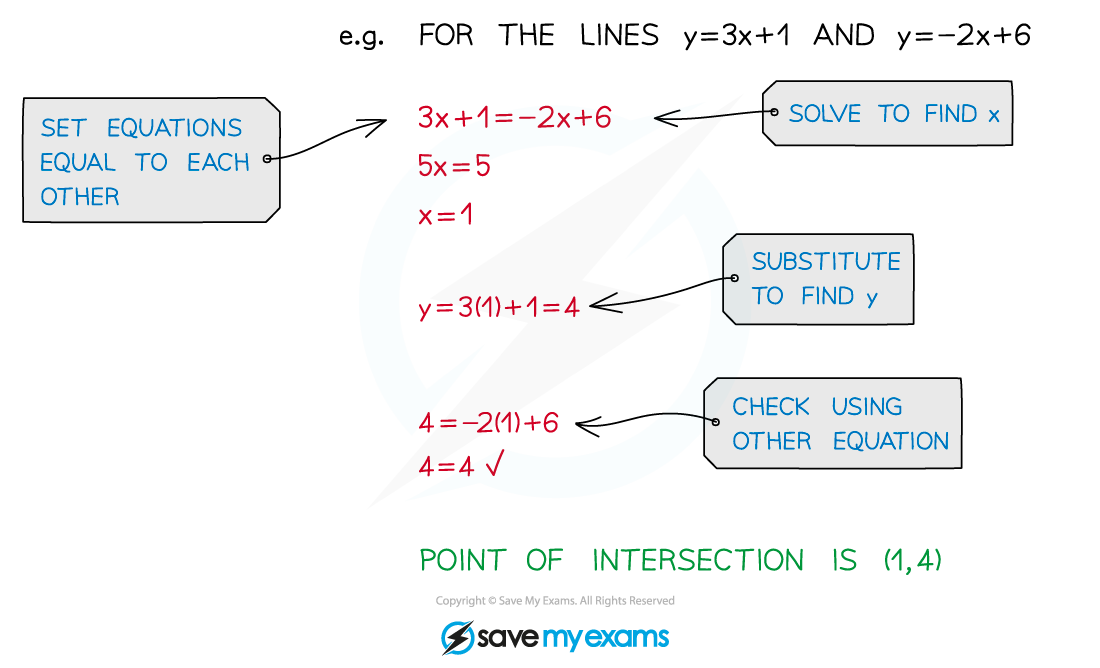#### Worked Example